Next: Choosing Run Parameters Up: Running the Program With Previous: Running the Program and

There are three types of adjustable parameters in parameters.h. The first are general parameters like the grid size, the second are model-specific parameters that have been copied in from a model file, and the third are parameters controlling the output of the program. We can't document the model-specific parameters because they are different for each model, but we describe here the other two groups.

The general parameters are

NDIMS
The number of dimensions. This can be set to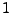,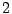, or.
N
The number of grid points per edge. The total number of points on the lattice is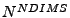.
nflds
The number of fields. Some models (e.g. the TWOFLDLAMBDA model described below) require a specific number of fields. In this case it is up to the model to check that the correct number of fields has been set when running that model. See section 4.2.2.
L
The size of the box in rescaled distance units. The volume of the box is.
dt
The size of the time step.
tf
The final time up to which the program should run.
seed
The seed for random number generation. This can be changed to produce two runs with different random initial distributions. (The random numbers are only used for initial conditions.)
initfield[]
An array of initial homogeneous field values in rescaled units. If there are fewer elements in the array than there are fields then all unspecified elements are set to zero. See section 6.3.1.
initderivs[]
An array specifying initial homogeneous field derivatives. Here too unspecified values are set to zero. See section 6.3.1.
expansion
The kind of expansion to use. This can be set to zero for no expansion (Minkowski space), one for a fixed power-law expansion, or two for a self-consistent calculation of the expansion coupled to the field evolution.
expansion_power
This parameter only has an effect if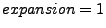. In this case expansion_power is the exponent of time in the fixed power-law expansion. Note that this exponent is given in physical, not rescaled units. For example it should be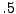orfor radiation or matter domination respectively. See section 6.2.3.
continue_run
This determines whether the program should start a new run () or look for a grid image file to continue an old run. If it does continue an old run this variable also determines whether new data will be appended to the old data files () or written in new files ().

The following are the parameters for controlling the output of the program. Note that all parameters that control a binary option (e.g. whether or not to calculate spectra) usefor yes andfor no. The output functions and the files they create are described in detail in section 5.

noutput_flds
The number of fields for which you want output (means, variance, spectra, etc.) to be generated. Set this to zero and the program will automatically generate output for all fields.
alt_extension
By default the program creates output files with the extension ``_0.dat" or ``_run_number.dat'' where ``run_number'' indicates whether or not the run is a continuation of a previous one. If you set this string to be anything other than empty then the program will use it as the extension for the output files instead.
noutput_times
The number of times during the run to call the save() function that calculates and outputs derived quantities. Means and variances of fields as well as the scale factor and its derivatives are calculated every time save() is called. Other quantities like spectra are calculated less frequently (see below).
print_interval
At evenly spaced intervals of clock time the program writes the current program time to an output file and, optionally, to the screen. This parameter determines the interval in seconds between these updates.
Whether or not to write time updates to the screen.
checkpoint_interval
How often (in program time units) to perform infrequent calculations and to save an image of the lattice. The infrequent calculations include spectra, components of energy, histograms, and slices.
store_lattice_times[]
An array of values giving times (in program units) at which to create new files for lattice images. By default the program creates a grid image file and stores the current version of the lattice in it every time it checkpoints. If there are no entries in this array then at the end of the run the only grid image will thus be of the lattice at the last time calculated. If there are entries in this array, however, then a separate file will be created with an image of the grid at each specified time, as well as at the end of the run. See section 5.9.
smeansvars
Whether or not to save means and variances of the fields. See section 5.2.
sexpansion
Whether or not to save the scale factor and its derivatives. This option is ignored unless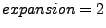. See section 5.3.
smodel
Whether or not to call a model-specific output function. See section 5.10.
t_start_output
This sets a time at which to start recording infrequent output. Actually the start time can be set for each output routine separately, but by default they are all set to begin at this time so that they can easily be changed all at once.
scheckpoint and tcheckpoint
Whether to save an image of the lattice (and when to start doing so).
sspectra and tspectra
Whether or not to save spectra of the fields (and when to start). See section 5.4.
senergy and tenergy
Whether or not to save the components of the energy density and a check of overall energy conservation (and when to start). See section 5.5.
shistograms and thistograms
Whether or not to generate histograms of the field values for each field (and when to start). See section 5.6.
nbins
The number of bins to use in the histograms
histogram_min and histogram_max
Upper and lower bounds for the histograms. If these are set equal to each other then the histograms automatically cover the range of current field values.
shistograms2d and thistograms2d
Whether or not to generate two-dimensional histograms of pairs of fields (and when to start). See section 5.7.
nbins2d
Default number of bins to use in each field direction.
nbins0
Number of bins to use in the first field direction.
nbins1
Number of bins to use in the second field direction.
histogram2d_min and histogram2d_max
Upper and lower bounds for the histograms. If these are set equal to each other then the histograms automatically cover the range of current field values.
hist2dflds[]
A list of the pairs of fields for which two-dimensional histograms should be generated. This list should always contain an even number of elements.
sslices and tslices
Whether or not to save the field values on a slice through the lattice (and when to start).
slicedim
The number of dimensions for the slice. If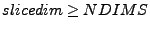then the whole lattice is output. Note that for a three dimensional lattice this could generate very large ASCII files.
slicelength
The number of points (in each dimension) to include in the slice.
sliceskip
The number of points between adjacent points in the slice. Set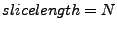and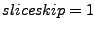to include the full slice.Next: Choosing Run Parameters Up: Running the Program With Previous: Running the Program and